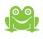# Pepperi Knowledge Center

1 comment

• Official commentWith the new and powerful Pepperi Rule Engine it can be done !

Load the Account Geo reference as TSAs (custom fields for the Account).

Use the Activity Submission coordinates (these are available within Pepperi for each Activity/Transaction) and calculate the distance from the Account.

Code that can be used to calculate the distance for example:

 function toRad(Value) {     /** Converts numeric degrees to radians */     return Value * Math.PI / 180; } var R = 6371000; /** Radius of planet Earth */   var P1 = toRad(AccountTSALAT); var P2 = toRad(SubmissionGeoCodeLAT); var P = toRad(SubmissionGeoCodeLAT -AccountTSALAT ); var L = toRad(SubmissionGeoCodeLNG - AccountTSALong ); var a = Math.sin(P/2) * Math.sin(P/2) + Math.cos(P1) * Math.cos(P2) * Math.sin(L/2)  * Math.sin(L/2); var c = 2 * Math.atan2(Math.sqrt(a), Math.sqrt(1-a)); var d = R * c; return d;

Now – for each activity you have the distance from the account.

Want even more ?

Add a rule that blocks specific activities (such as "Visit") from being created if the distance is >  XXX meters

The Pepperi Rule Engine is a feature available in the Ultimate Plan and may require Pepperi services to implement.

This is just one example of the many possibilities to implement rules that apply checks and constraints on your Transactions and Activities.

Merav Bitton